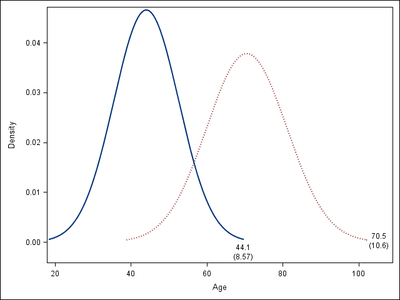## About inset statement: proc sgplot vs. proc univariate

I apply the proc sgplot to put two PDF curves on one graph, like the code below:

proc sgplot data=have;
density var1 / legendlabel="var1" lineattrs=(pattern=solid);
density var2 / legendlabel="var2" lineattrs=(pattern=dot);
xaxis label="value";
run;

then, I try to add the mean and std for both curves, which can be done in proc univariate by the inset statement:

inset normal(mu sigma);

I think that the inset statement does not work in the same way in proc sgplot as it does in proc univariate. Any idea to let the above proc sgplot also output mean and std for both PDF curves? Thanks!

1 ACCEPTED SOLUTION

Accepted SolutionsPGStats
Opal | Level 21

## Re: About inset statement: proc sgplot vs. proc univariate

Here is an example of one of many ways to display these statistics:

``````proc univariate data=sashelp.heart noprint;
var ageatstart ageatdeath;
output out=ageStats mean=muStart muDeath std=stdStart stdDeath;
run;

data _null_;
set ageStats;
str = catt(put(muStart,4.1),"/(",put(stdStart,4.2),")");
call symputx("startLabel",str);
str = catt(put(muDeath,4.1),"/(",put(stdDeath,4.2),")");
call symputx("deathLabel",str);
run;

proc sgplot data=sashelp.heart;
density ageatstart / legendlabel="Start Age"
curvelabel="&startLabel" curvelabelpos=max splitchar="/" splitjustify=center
lineattrs=(pattern=solid);
density ageatdeath / legendlabel="Death Age"
curvelabel="&deathLabel" curvelabelpos=max splitchar="/" splitjustify=center
lineattrs=(pattern=dot);
xaxis label="Age";
run;``````PG
4 REPLIES 4

## Re: About inset statement: proc sgplot vs. proc univariate

Yes, you are exactly right. PROC SGPLOT is primarily a graphical procedure. It does not contain the same analytical capabilities as ROC UNIVARIATE.  In particular, the INSET statement requires that you specify the text to display, which means you probably want to use PROC UNIVARIATE to fit the distribution to the data. Let's say that PROC UNIVARIATE displays a table that states that the parameters are mu=1.2 and sigma=3.4. Then you might use the following INSET statement in PROC SGPLOT:

inset ("mu" = "1.2" "sigma" = "3.4") / border;PGStats
Opal | Level 21

## Re: About inset statement: proc sgplot vs. proc univariate

Here is an example of one of many ways to display these statistics:

``````proc univariate data=sashelp.heart noprint;
var ageatstart ageatdeath;
output out=ageStats mean=muStart muDeath std=stdStart stdDeath;
run;

data _null_;
set ageStats;
str = catt(put(muStart,4.1),"/(",put(stdStart,4.2),")");
call symputx("startLabel",str);
str = catt(put(muDeath,4.1),"/(",put(stdDeath,4.2),")");
call symputx("deathLabel",str);
run;

proc sgplot data=sashelp.heart;
density ageatstart / legendlabel="Start Age"
curvelabel="&startLabel" curvelabelpos=max splitchar="/" splitjustify=center
lineattrs=(pattern=solid);
density ageatdeath / legendlabel="Death Age"
curvelabel="&deathLabel" curvelabelpos=max splitchar="/" splitjustify=center
lineattrs=(pattern=dot);
xaxis label="Age";
run;``````PG

## Re: About inset statement: proc sgplot vs. proc univariate

Thank you all for your kindly help

## Re: About inset statement: proc sgplot vs. proc univariate

I add  name="curve1" and name="curve2" in those two  density statements, separately. So that legendlabel can also display.

Discussion stats
• 4 replies
• 1291 views
• 2 likes
• 3 in conversation